# Nodal Analysis Circuit for Electric Circuit and ExamplesNodal analysis circuit finds the unknown voltage drops around a circuit between different nodes that provide a common connection for two or more circuit components.

Kirchhoff’s current law (KCL) and Kirchhoff’s voltage law (KVL) are almost sufficient for analyzing an electric circuit with less complexity. What will we get from KVL or KCL for more complicated circuits with more complex branches, nodes, and elements? You will get a lot of equations you need to solve.

What will we do then? It will be time-consuming if we have to use elimination and substitution for more than 4 equations.

This is where we will use three powerful techniques for circuit analysis :

We will leave the mesh analysis for a later post. Right now, let’s focus on Node Voltage Analysis or Nodal Analysis Circuit.

As the name implies, we will use the node voltages method in respect to the Ground, hence it is called Node Voltage Analysis.

## What is Nodal Analysis Circuit

Nodal analysis circuits complement each other with mesh analysis circuits. The nodal analysis circuit uses the first Kirchhoff’s law, the Kirchhoff’s current law (KCL). Like we mentioned above, the name implies that we use node voltages and use it along with the KCL.

Nodal analysis needs us to calculate node voltages in each node with respect to the ground voltage (reference node), hence it is called the node-voltage method.

Nodal analysis is based on a systematic application of Kirchhoff’s current law (KCL). With this technique, we will be able to analyze any linear circuit.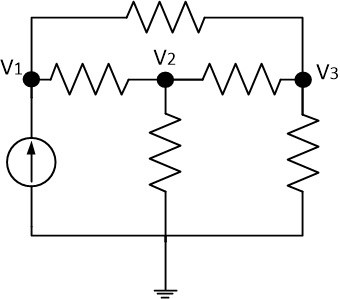What do you need to prepare before using this method? Keep in mind that we will get ‘n-1’ equations, where n is the number of nodes including the reference node. Using this circuit analysis method means we will focus on node voltages in the circuit.

Nodal analysis circuit properties:

1. Nodal analysis circuit uses the Kirchhoff’s current law (KCL)
2. For the ‘n’ nodes (including reference node) there will be ‘n-1’ independent nodal voltage equations
3. Solving all the equations will grant us the node voltages value
4. The number of nodes (except non-reference nodes) is equal to the number of the nodal voltage equation we can get.

## What is Node Voltage

Before moving on, let us define ‘what is node voltage‘. Node voltages are the potential difference between two nodes of a circuit.

Notice at the circuit above, where v1, v2, and v3 are the node voltages, connecting the corresponding node with element/s and another node.

Not only that, but we also need to define a reference node (ground), hence this node is always called a ground node. Thus, this node voltage is 0 V.

## Types of Nodes in Nodal Analysis Circuit

We have read a lot about node voltage. But what node voltage actually is? Node voltage means the potential difference (voltage) between two nodes where the element or branch is present. The nodal analysis provides us with a mathematical equation for every non-reference node where the sum of the currents in a node is zero.

There are two types of node:

1. Reference nodes: reference nodes are the ground node
2. Non-reference nodes: the node voltages used for solving the circuit (v1, v2, v3,… , vn)

### Types of reference nodes

Let’s take a look at the figure below:

There are two types of reference nodes:
1. Earth ground: Figure.(1a) and (1b)
2. Chasis ground: Figure.(1c)

You can choose one of them while doing the nodal analysis circuit.

## Nodal Analysis Circuit Procedure

The first step is to define a node as the reference or datum node or the ground node. The reference node is usually called the ground since it is assumed to have zero potential.

The symbol of the reference node is shown in Figure.(1). Earth ground is shown in Figures.(1a) and (1b) and chassis ground is shown in Figure.(1c).

After we get the reference node, we assign voltage designations to reference nodes.

Take a look at Figure.(2a) where node 0 is the reference node (v = 0), while nodes 1 and 2 are assigned with voltages v1 and v2 respectively.

Remember, node voltages are defined with respect to the reference node. As shown in Figure.(2a), each node voltage is the voltage rise from the reference node to the non-reference node or simply of the voltage node to the reference node.

The second step is to apply KCL to each non-reference node in the circuit. To reduce the complexity of variables, Figure.(2a) is redrawn in Figure.(2b), where we use i1, i2, and i3 as the currents flowing through resistors R1, R2, and R3 respectively.

At node 1 we apply KCL and gives

(1)At node 2 gives

(2)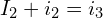We apply Ohm’s law to express the unknown value of i1, i2, and i3 in terms of node voltages.

Since the resistance is a passive element, using the passive sign convention, the current must always flow from higher potential to a lower potential.

Current flows from a higher potential to a lower potential in a resistor.

We can use this principle as,

(3)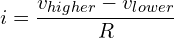We obtain from Figure.(2b),

(4)Substituting Equations.(4) in (1) and (2) results

(5)(6)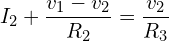Substituting with conductances, Equations.(5) and (6) become

(7)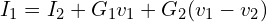(8)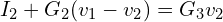The third step is solving with node voltages. Applying KCL to n – 1 non reference node, we obtain n – 1 simultaneous equations such as Equations.(5) and (6) or (7) and (8).

For the circuit in Figure.(2) we solve Equations.(5) and (6) or (7) and (8) to get the node voltages v1 and v2 using any standard method such as substitution method, elimination method, Cramer’s rule, or matrix inversion.

For example Equations.(7) and (8) will use matrix form as

(9)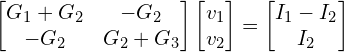which can be solved to get v1 and v2.

Please take note that we will find the resistor, voltage source, and current source in the circuit. There will be special treatment to a voltage source and current source. If the steps above are quite complex, you may want to read the short explanation below how to treat each element:

### Nodal analysis with resistor

This one is the most basic because almost every circuit will contain at least one resistor. Assume that we have a resistor between two nodes and the current flows from node V1 to V2:And then we get the equation: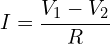That is the equation for a resistor between a node.
What if node 2 is ground (reference node) as shown below?The equation will be the same as above, but we will set the V2 to 0.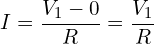### Nodal analysis with voltage source

It is often that a branch consists of a voltage source in a resistor just as shown below: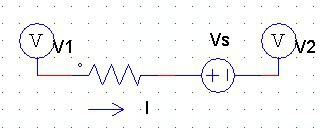We need to take care of the voltage source polarity. From the figure above, the voltage source positive polarity is facing against V1 and I. It means the current from the voltage source is flowing against I and V1. The equation will be: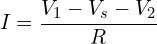If the voltage source is facing right, it means the current I will be summed with the current from Vs.Hence,If the V2 is a reference node, you just need to set the V2 to 0 like before.

### Nodal analysis with current source

We use nodal analysis to get work with KCL in which, acquiring the current equation using known node voltages. What will happen if there is a current source? This will make our equation simpler. First, look at the figure below.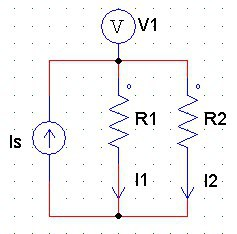We set both I1 and I2 leaving the node V1 while the current source Is is entering node V1. The equation will be: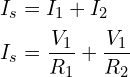If the current source Is leaving the node V1, the equation will be:## Nodal Voltage Analysis Examples

For better understanding, let us review some examples below :
1.Calculate the node voltages in the circuit in Figure.(3a)

Solution :

Consider Figure.(3b) where the circuit in Figure.(3a) has been prepared for nodal analysis. The currents have been selected for KCL except for the branches with current sources.

The labelling of the current is arbitrary but consistent. (Consistent means if, for example, i2 enters the 4 Ω resistor from the left-hand side, i2 must leave the resistor from the right-hand side).

The reference node is selected and the node voltages v1 and v2 are now determined.

At node 1, applying KCL and Ohm’s law givesMultiplying each term in the last equation by 4, we obtainor

(1.1)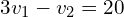At node 2, we do the same and get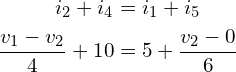Multiplying each term by 12 results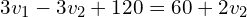or

(1.2)Now we have two simultaneous (1.1) and (1.2) and then we can solve using any method to get v1 and v2.

Method 1

Using the elimination method we add (1.1) and (1.2) gives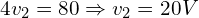Substituting the result above with (1.1) gives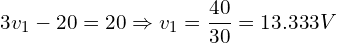Method 2

Use Cramer’s rule, we put (1.1) and (1.2) to matrix form as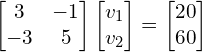The determinant isWe now obtain the voltages as2.Determine the voltages at the nodes in Figure.(4a)

Solution :

In this example, we will need three nonreference nodes instead of only two. We assign three nodes as can be seen in Figure.(4b)

At node 1,Multiplying by 4 and rearranging terms, we get

(2.1)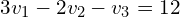At node 2,Multiplying by 8 and rearranging terms, we get

(2.2)At node 3,Multiplying by 8, rearranging terms, and dividing by 3, we get

(2.3)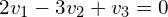We now will use the elimination method, we add (2.1) and (2.3)

(2.4)(2.5)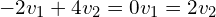Substituting (2.5) into (2.4) we getFrom (2.3), we getThus,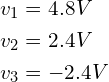Next we will learn about supernode voltage analysis.

### What is meant by nodal analysis?

Nodal analysis circuit is a method of calculating the node voltage between nodes to get the branch current.

### How do you do a nodal analysis?

1. Determine all the nodes
2. Choose a reference node (ground)
3. Calculate all the node voltages
4. Write and solve all the KCL equations

### What are the limitation of nodal analysis?

While it is a powerful method, nodal analysis circuit needs more complex execution while there is voltage source and dependent-sources.